# What Are Functions?

What Are Functions?

Functions are the part of calculus in mathematics. Before begin with indepth learning about function, let us clear our concepts of range, domain, and codomain.

Some of the most important aspects of a function include Domain, Range, and Codomain. These three basic concepts are the foundation for defining any function. In fact, these may as well make or break an entire function. Hence it is important to have knowledge about each.

Domain is defined as the set of all the values that the function can input while it can be defined.

Range are all the values that come out as output of the function involved.

Codomain is the set of values that have the potential of coming out as outputs of a function.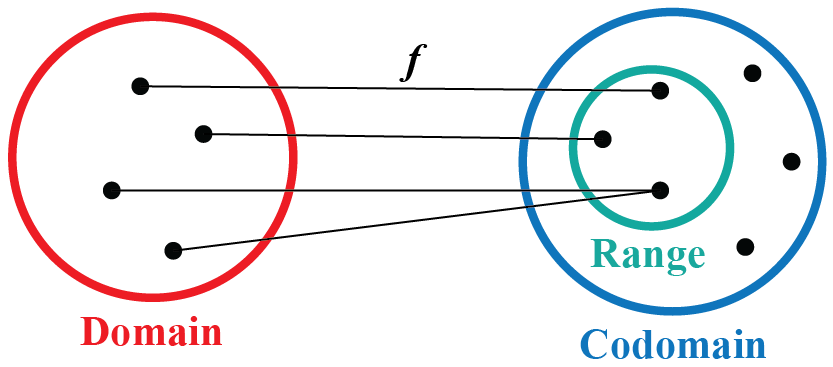In this mini lesson, we will explore the world of functions. We will walk through the answers to the questions like what are functios, how to represent a function, what are the conditions for a function, how to solve a function, types of function, and various graphs of functions along with solved examples and interactive questions.

## Lesson Plan

 1 What Are Functions? 2 Important Notes on What Are Functions 3 Solved Examples on What Are Functions 4 Interactive Questions on What Are Functions 5 Challenging Question on What Are Functions

## What Are Functions?

In mathematics, a function means a correspondence from one value x of the first set to another value y of the second set. This correspondence can be of the following four types. But each correspondence is not a function.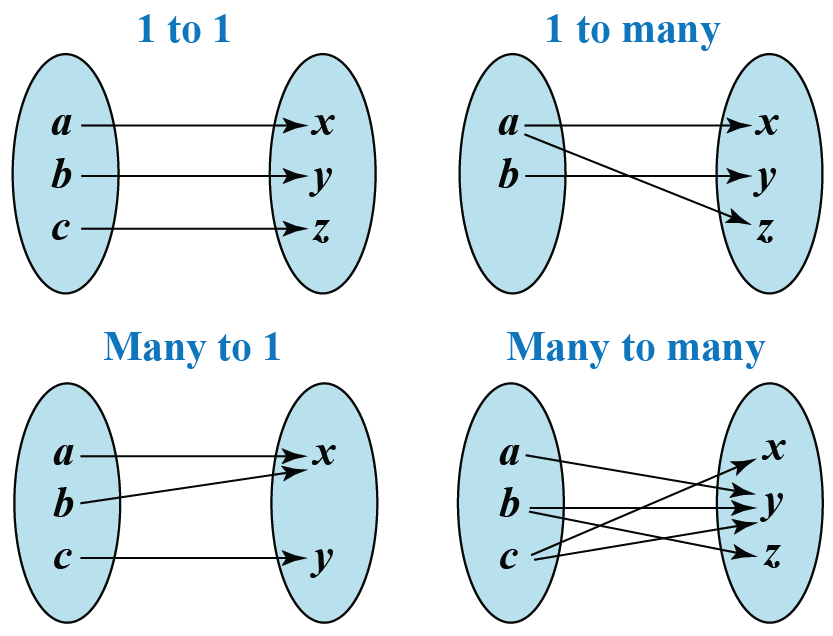In the above figure, only 1 – 1 and many to one are examples of a function because no two ordered pairs have the same first component and all elements of the first set are linked in them.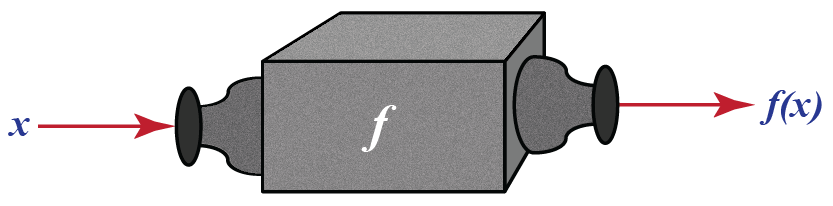So we say that in a function one input can result in only one output. If we are given any x then there is one and only one y that can be paired with that x.
The following diagram depicts a function: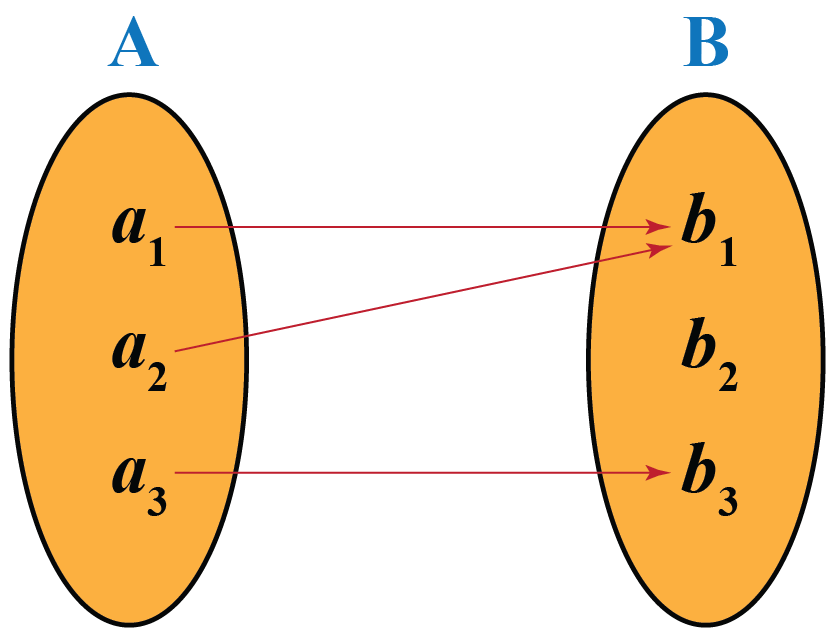A function is a specific type of relation. Any relation may have more than one output for any given input. For example:-

1. The temperature on any day in a particular city.
2. The number of calories based on your food intake.
3. The number of places you can visit with one gallon in your car's fuel tank
4. The number of sodas coming out of a vending machine depending on how much money you insert.
5. The amount of carbon left in a fossil after a certain number of years.
6. The height of a person at a specific age.

But for a function, every x in the first set should be linked to a unique y in the second set. So examples 1, 2, and 3 above are not functions. And examples 4, 5, and 6 are functions.

### Representation of Functions

"f(x) = x2 " is the general manner to represent  function.

It is said as f of x is equal to x square.

### Special Rules:

• Function must work for all the possible input value
• With each input value it must have one relationship only.

## How To Solve a Function?

Let us solve an example to understand how to solve a function.

Given two functions: $$\text f = {(-1, 1), (0, 2), (4, 5)}$$ and $$\text g = {(1, 1), (2, 3), (7, 9)}$$, find $$( \text g \circ \text f)$$ and determine its domain and range.

Here,

\begin{align*} \text g ( \text f ( x)) &= \text g \circ \text f \\ &= (\text g \circ \text f)( -1 ) \\ &= \text g [ \text f (-1) ] \\ &= \text g(1) \\ &= 1 \end{align*}

Similarly,

\begin{align*} \text g ( \text f ( x)) &= \text g \circ \text f \\ &= (\text g \circ \text f)( 0 ) \\ &= \text g [ \text f (0) ] \\ &= \text g(2) \\ &= 3 \end{align*}

Also,

\begin{align*} \text g ( \text f ( x)) &= \text g \circ \text f \\ &= (\text g \circ \text f)( 4 ) \\ &= \text g [ \text f (4) ] \\ &= \text g(5) \\ &= \text {undefined} \end{align*}

Hence,

$$( \text g \circ \text f ) = {(-1, 1), (0, 3)}$$

Therefore, Domain: {-1, 0} and Range: {0, 3}

\begin{align*} \therefore( \text g \circ \text f ) &= {(-1, 1), (0, 3)} \\ \text {Domain} &= (-1,0) \\ \text {Range} &= (0, 3) \end{align*}

## Identify Types of Functions With Graph

The various types of functions are:

### Identity Function

Identity function is the type of function which gives the same input as the output.

It is expressed as,

$$f(x) = x$$, where $$x \in \mathbb{R}$$

For example, $$f(3) = 3$$ is an identity function.

The following figure shows the plot of an identity function.### Constant Function

Constant function is the type of function which gives the same value of output for any given input.

It is expressed as,

$$f(x) = c$$, where $$c$$ is a constant

For example, $$f(x) = 2$$ is a constant function.

The following figure shows the plot of a constant function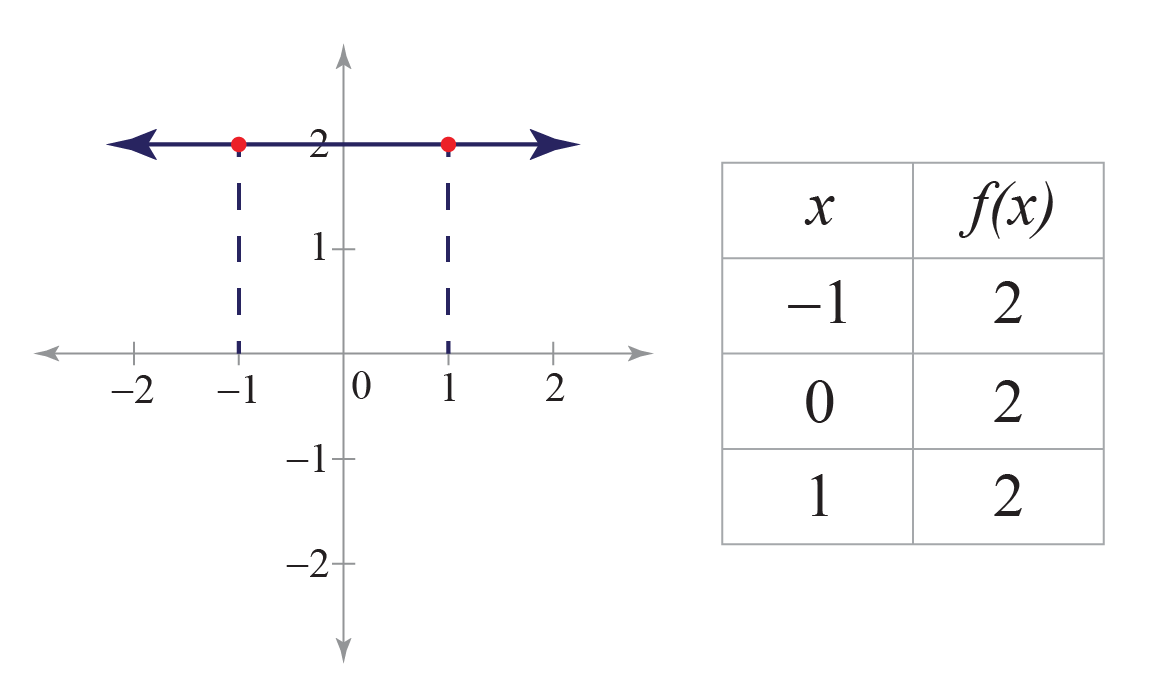### Polynomial Function

Polynomial function is a type of function which can be expressed as a polynomial.

It is expressed as,

$$f(x) = a_{n}x^{n} + a_{n-1}x^{n-1} +....... + a_{0}x^{0}$$

For example, $$f(x) = 2x+5$$ is a polynomial function.

The following figure shows the plot of a polynomial function

$f\left( x \right) = {x^3} - 6{x^2} + 11x - 6$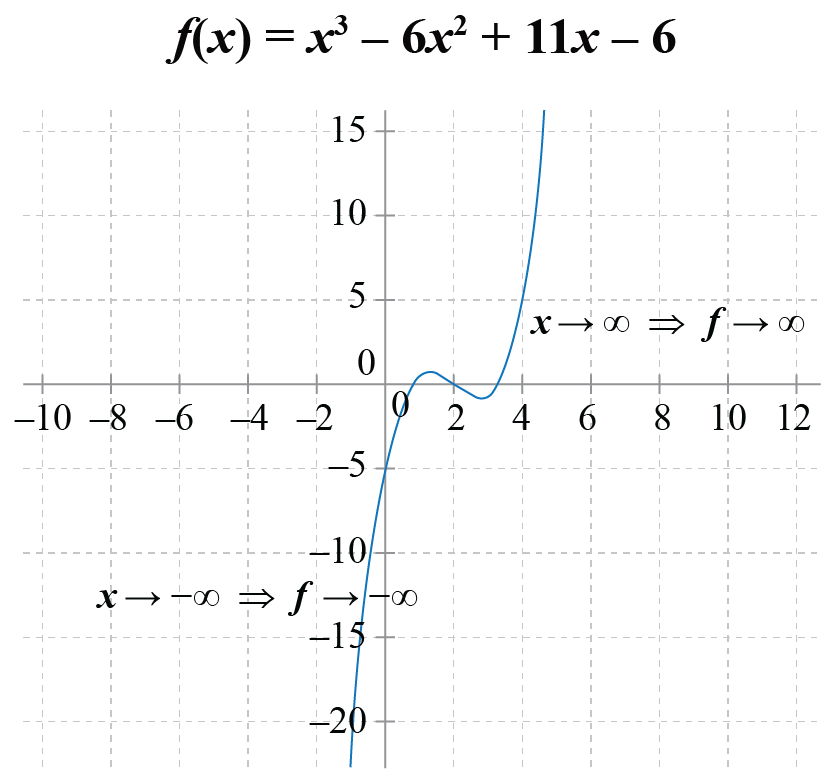Note that the scale of the two axes is different.

### Quadratic Function

Quadratic function is a type of function which has the highest power 2 in the polynomial function.

It is expressed as,

$$f(x)=a(x-h)^{2}+k$$.

For example, $$f(x) = x^2 + 1$$ is a quadratic function.

The following figure shows the plot of quadratic function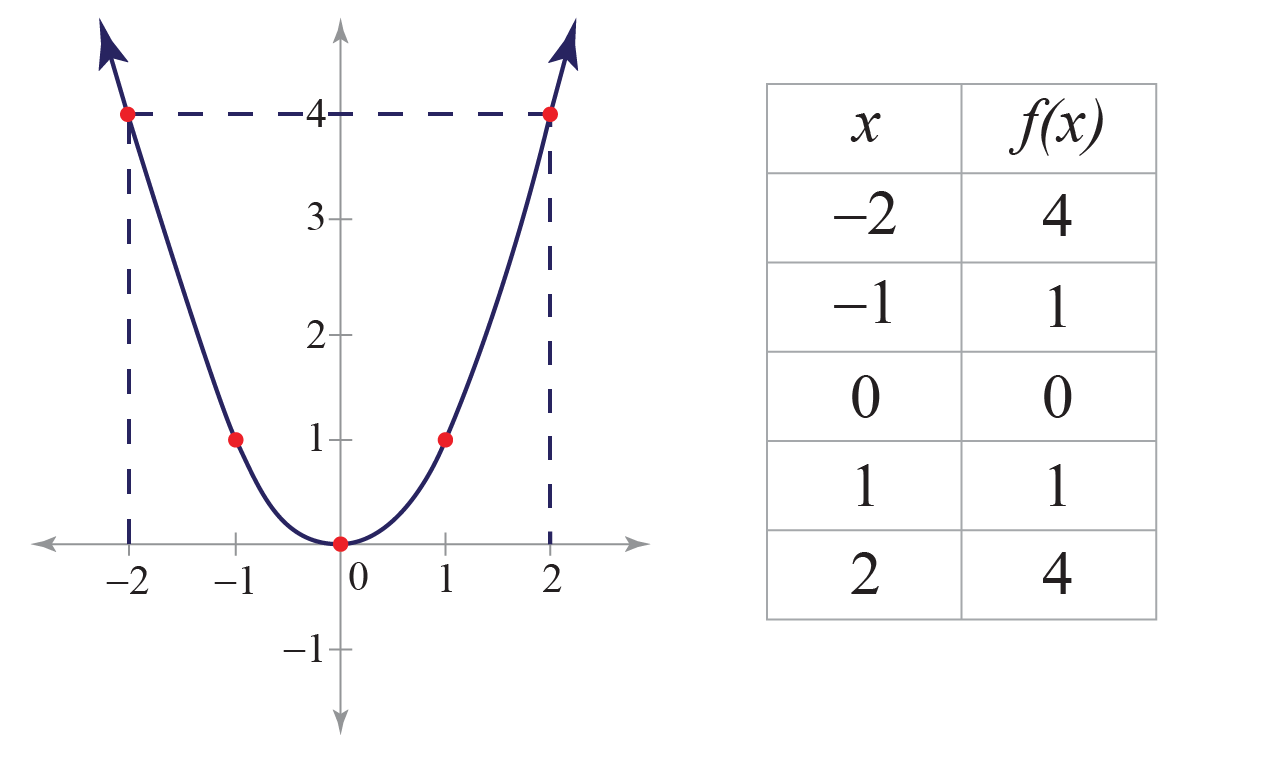### Cubic Function

Cubic function is a type of function which has the highest power 3 in the polynomial function.

It is expressed as,

$$f(x) = a_{1}x^{3} + a_{2}x^{2} + a_{3}x + a_{4}$$

For example, $$f(x) = x^3 + 4$$ is a cubic function.

The following figure shows the plot of a cubic function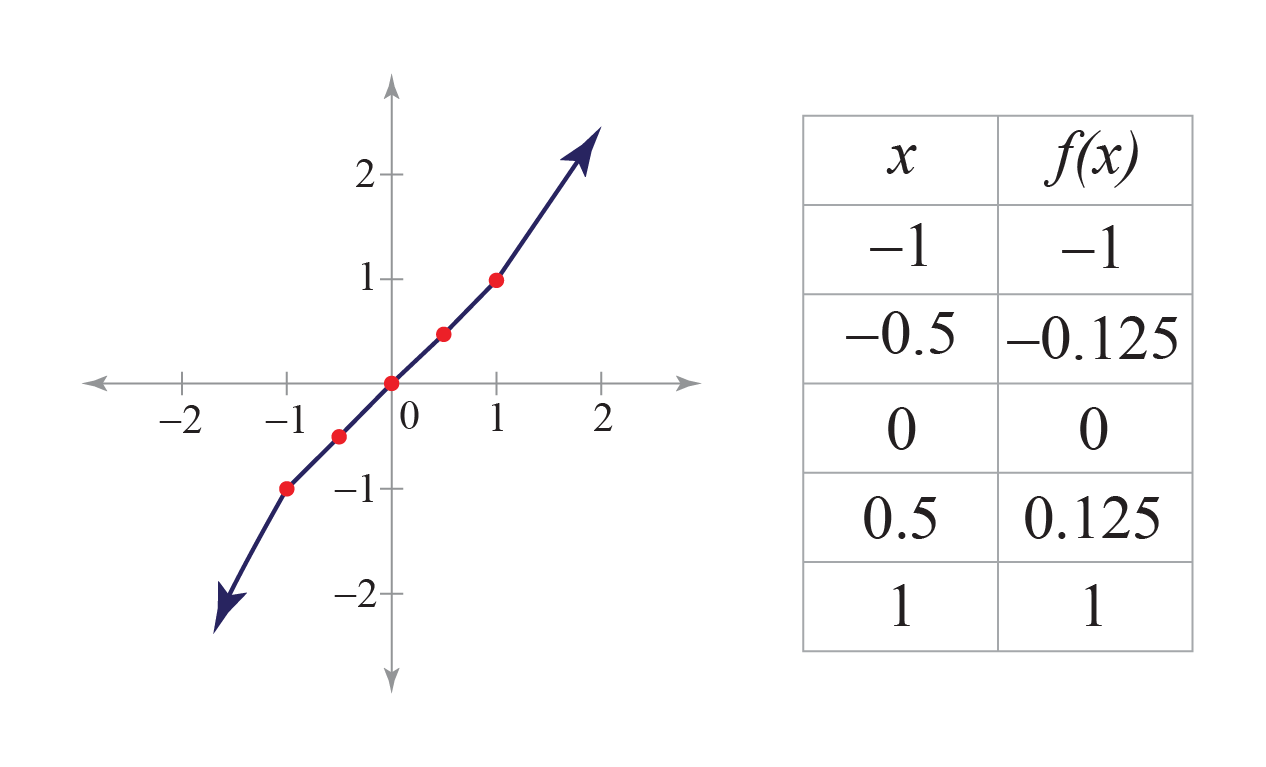### Rational Function

Rational function is a type of function which is derived from the ratio of two polynomial functions.

It is expressed as,

$$f(x) = \frac{P(x)}{Q(x)}$$, such that P and Q are polynomial functions of $$x$$ and $$Q(x)\neq0$$

For example, $$f(x) = \frac{x^2 + 2x + 1}{x^2 - 4}$$ is a rational function.

The following figure shows the plot of a rational function.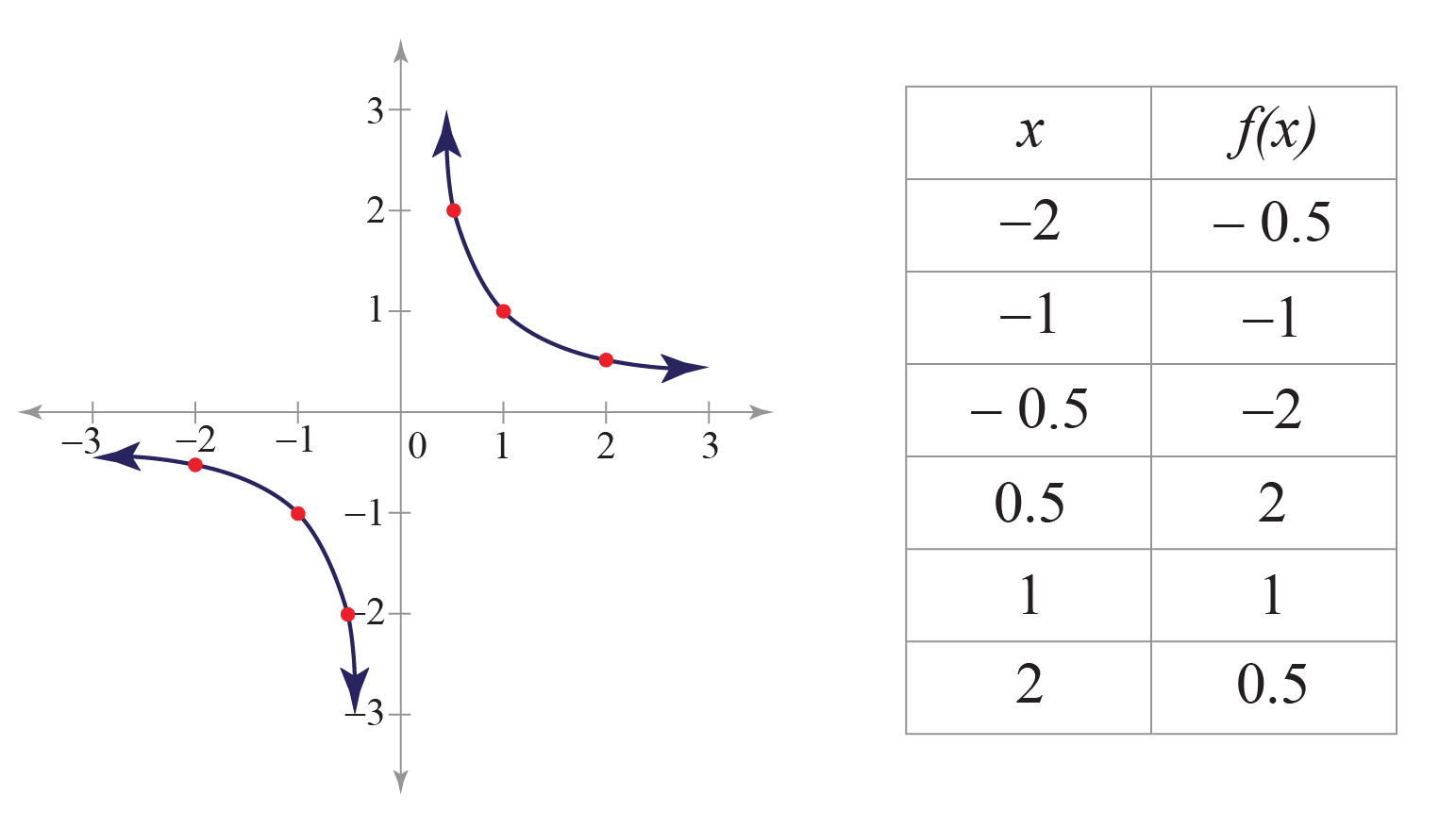### Modulus Function

Modulus function is a type of function which gives the absolute value of a number by giving its magnitude.

It is expressed as,

$$f(x) = |x|$$

It can further be defined as:

$$\begin{equation*} f(x) = \begin{cases} x & x \geq 0\\ -x & x < 0\\ \end{cases} \end{equation*}$$

The following figure shows the plot of a modulus function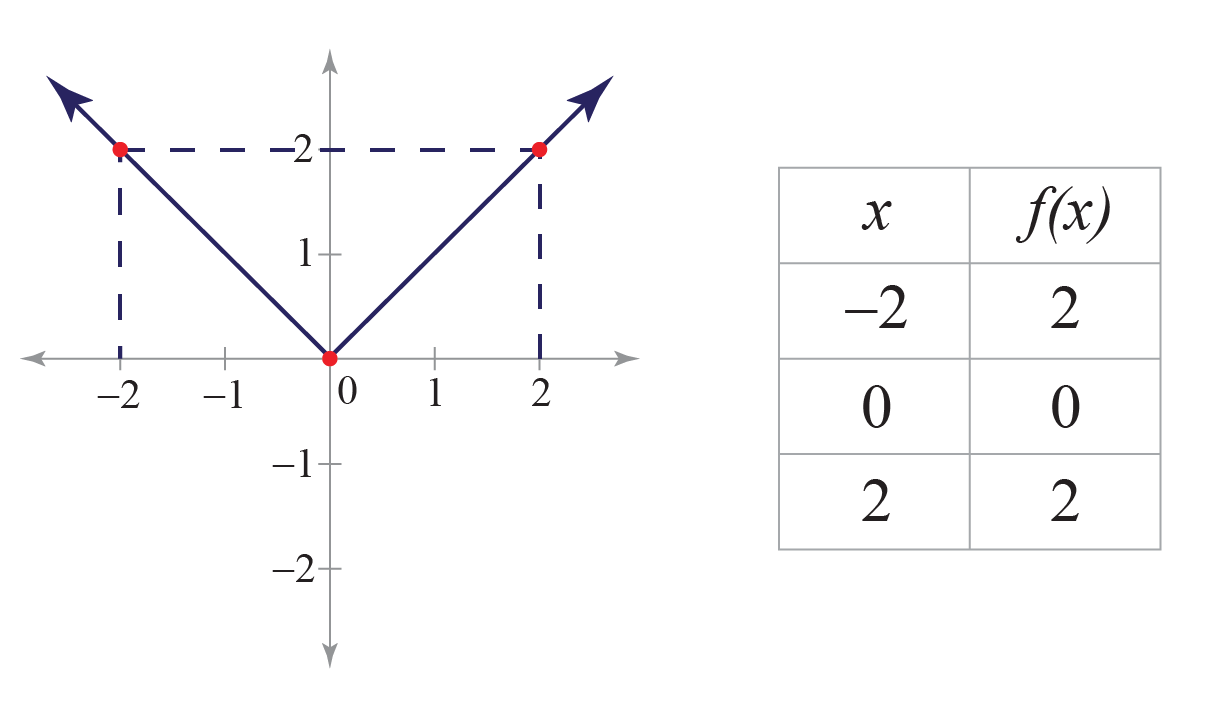More Important Topics
Numbers
Algebra
Geometry
Measurement
Money
Data
Trigonometry
Calculus